# Week 1: Square Dance

Week 1: Square Dance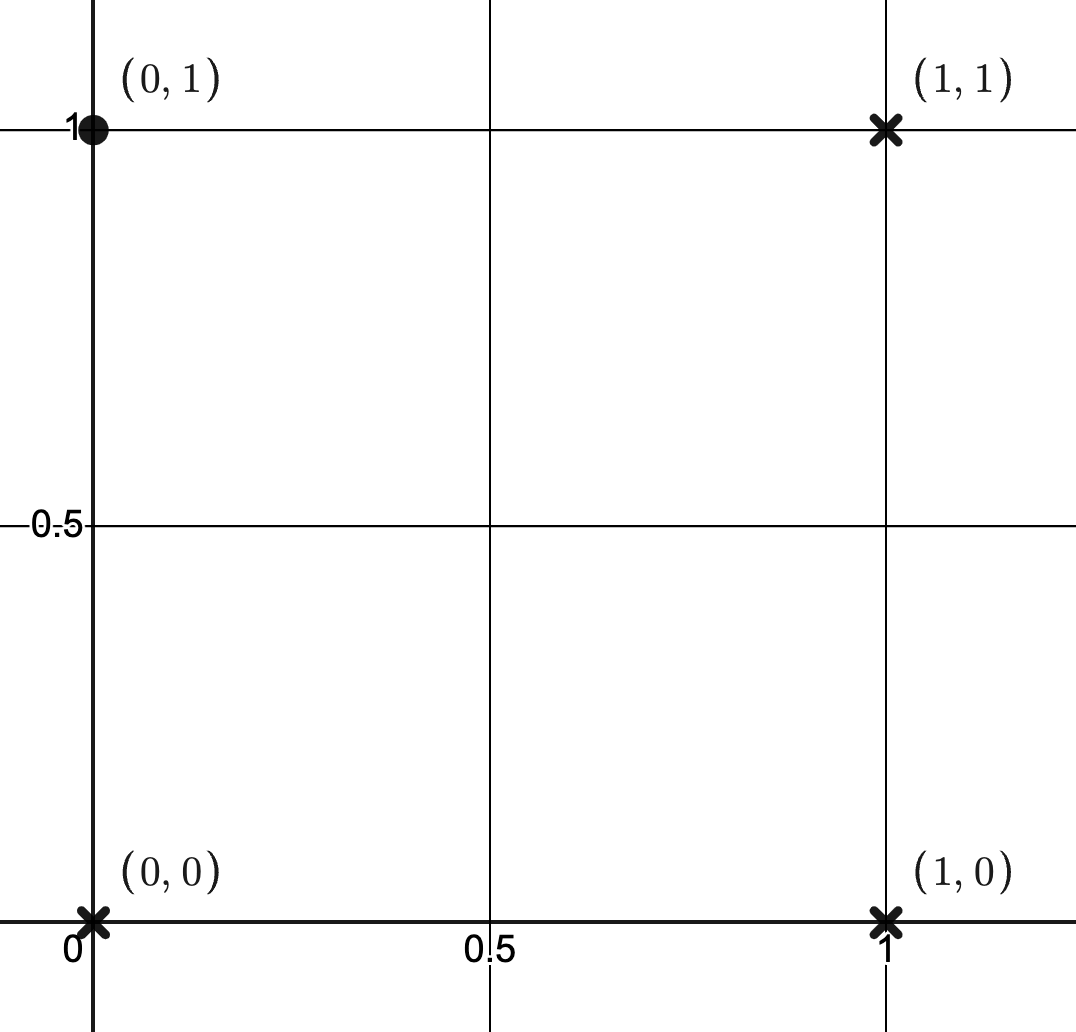This puzzle is as follows:

For the unit square shown above with vertices at (0,0), (1,0), (0,1) and (1,1), how many different types of functions can you find that go through at least 2 points?

Teacher notes:

Level of difficulty:  Accessible for strong SL and all HL students.

Syllabus knowledge required:  Functions and transformations and/or regression techniques.

This would be a good starter which could either be done as a non GDC exercise, or using Desmos regression depending on what syllabus point you intend to support.  It would be a useful exercise to support the coursework.

Solutions:

There are clearly an unlimited number of solutions.  Here are a few: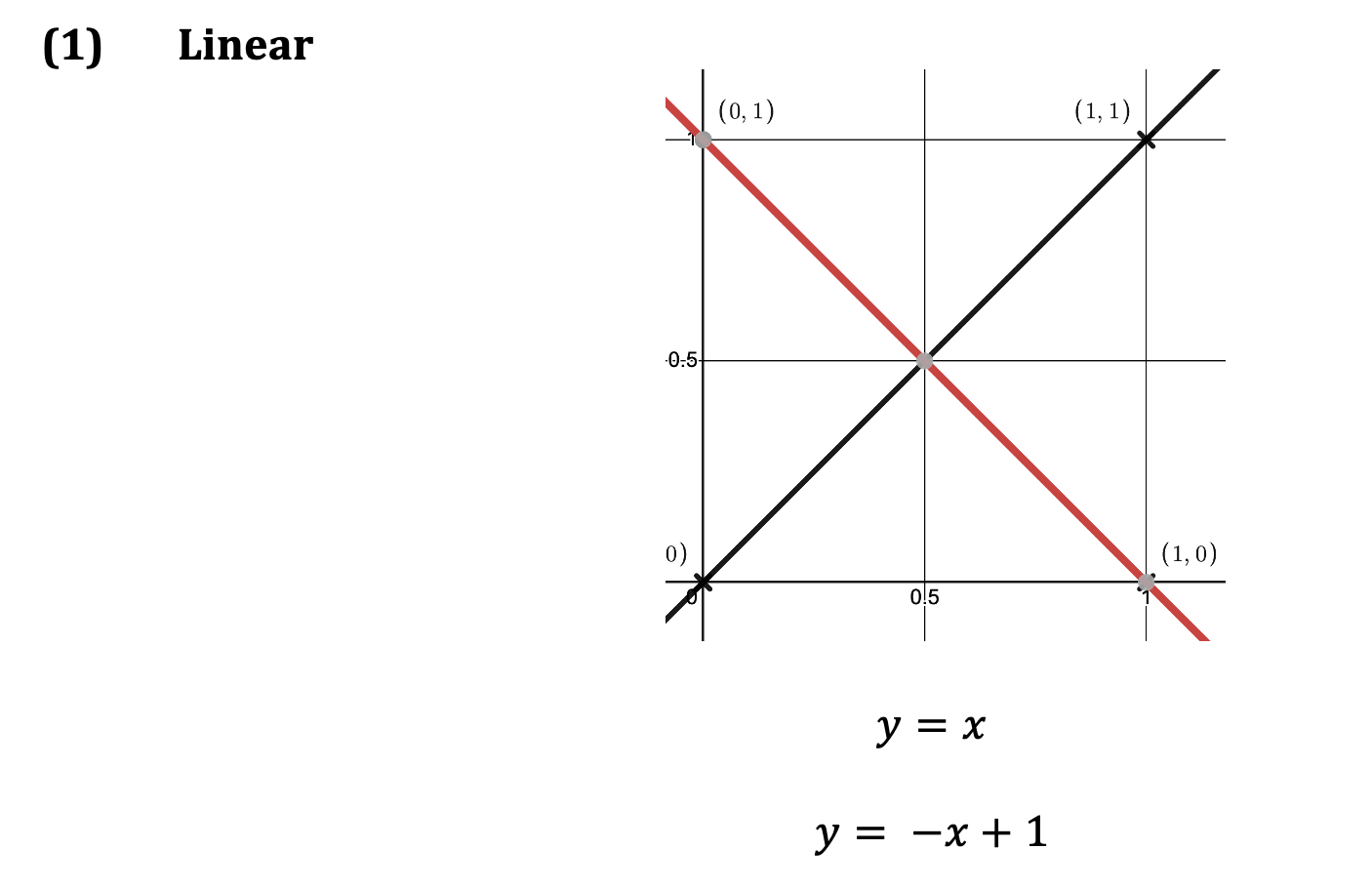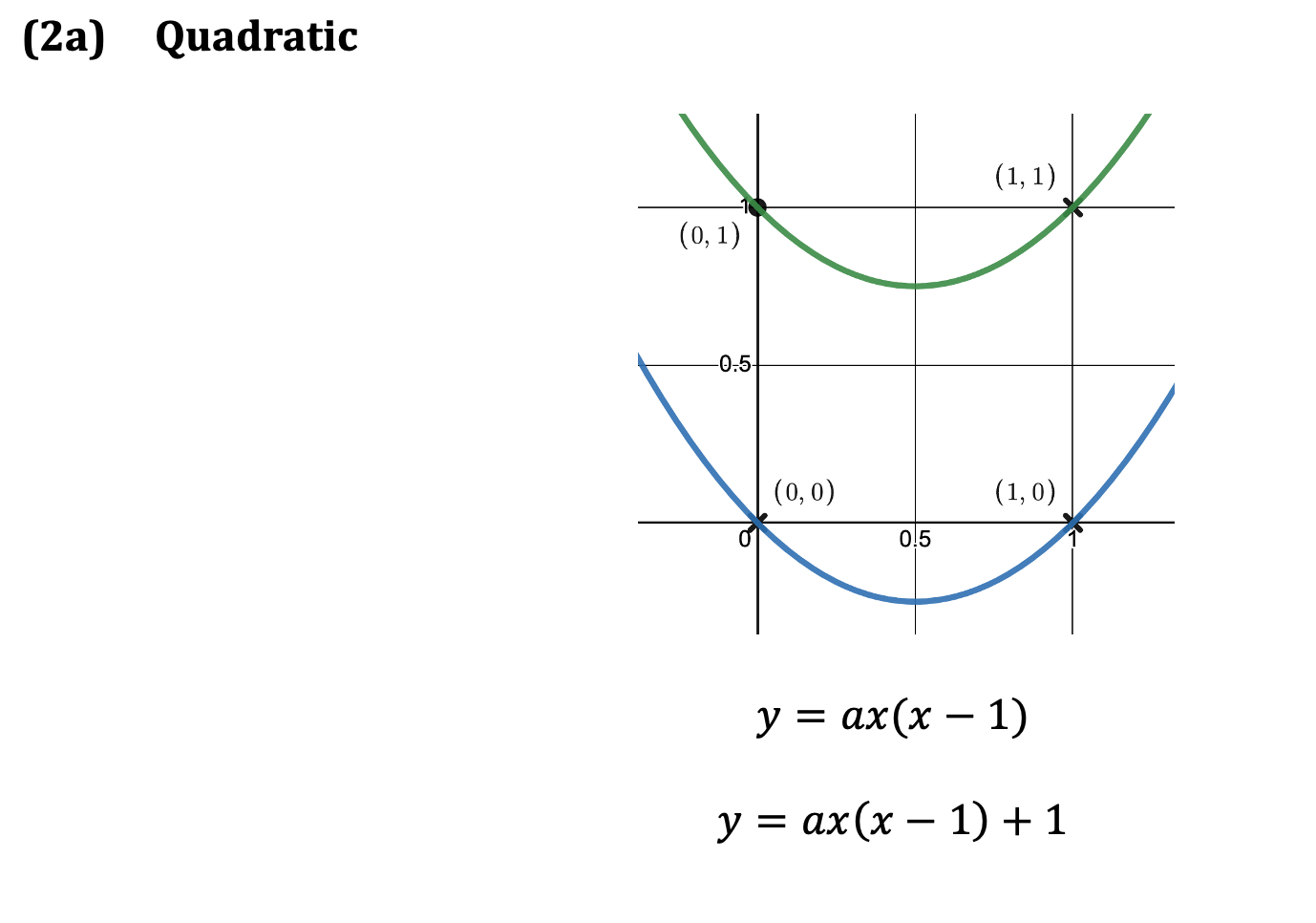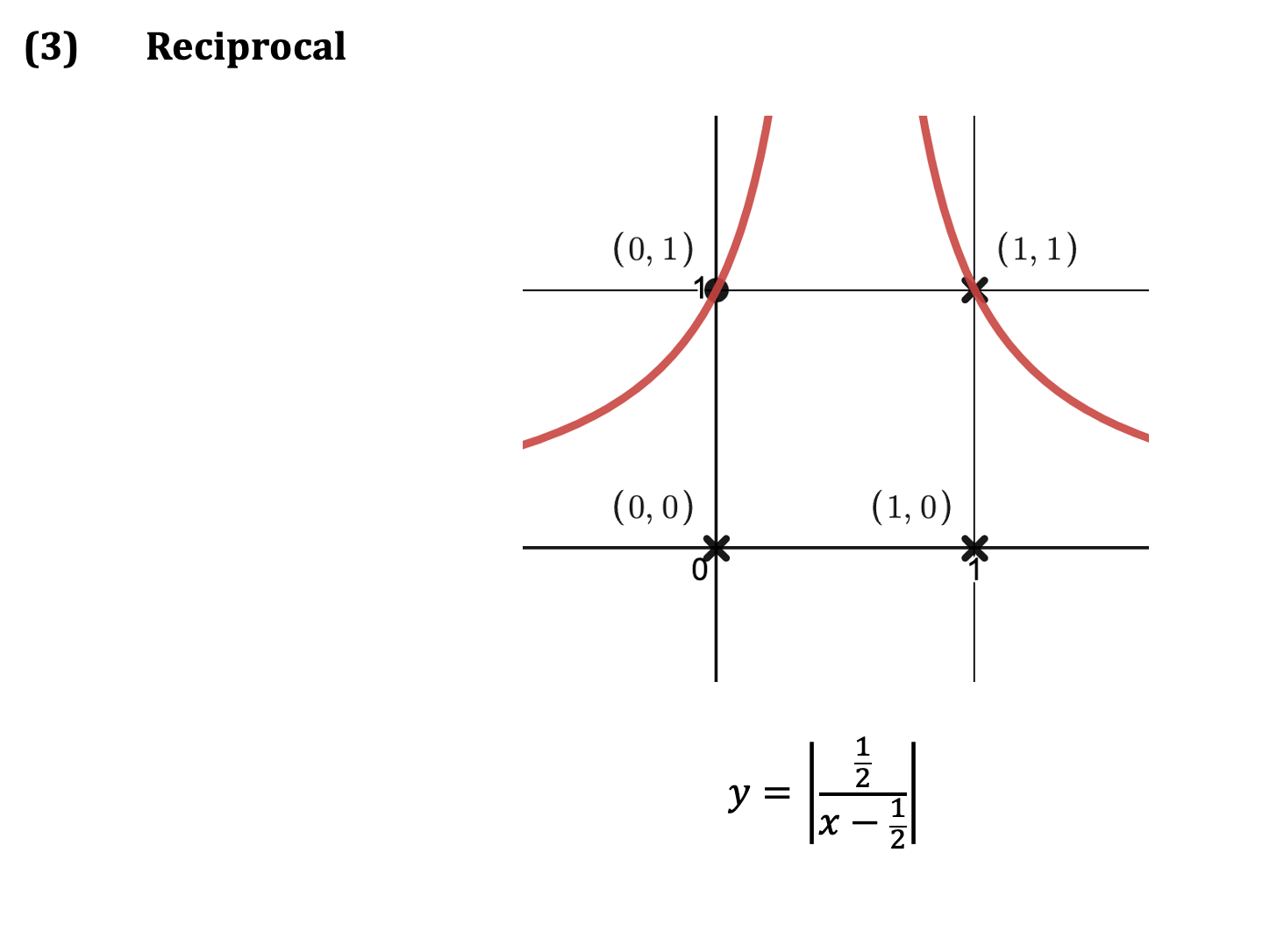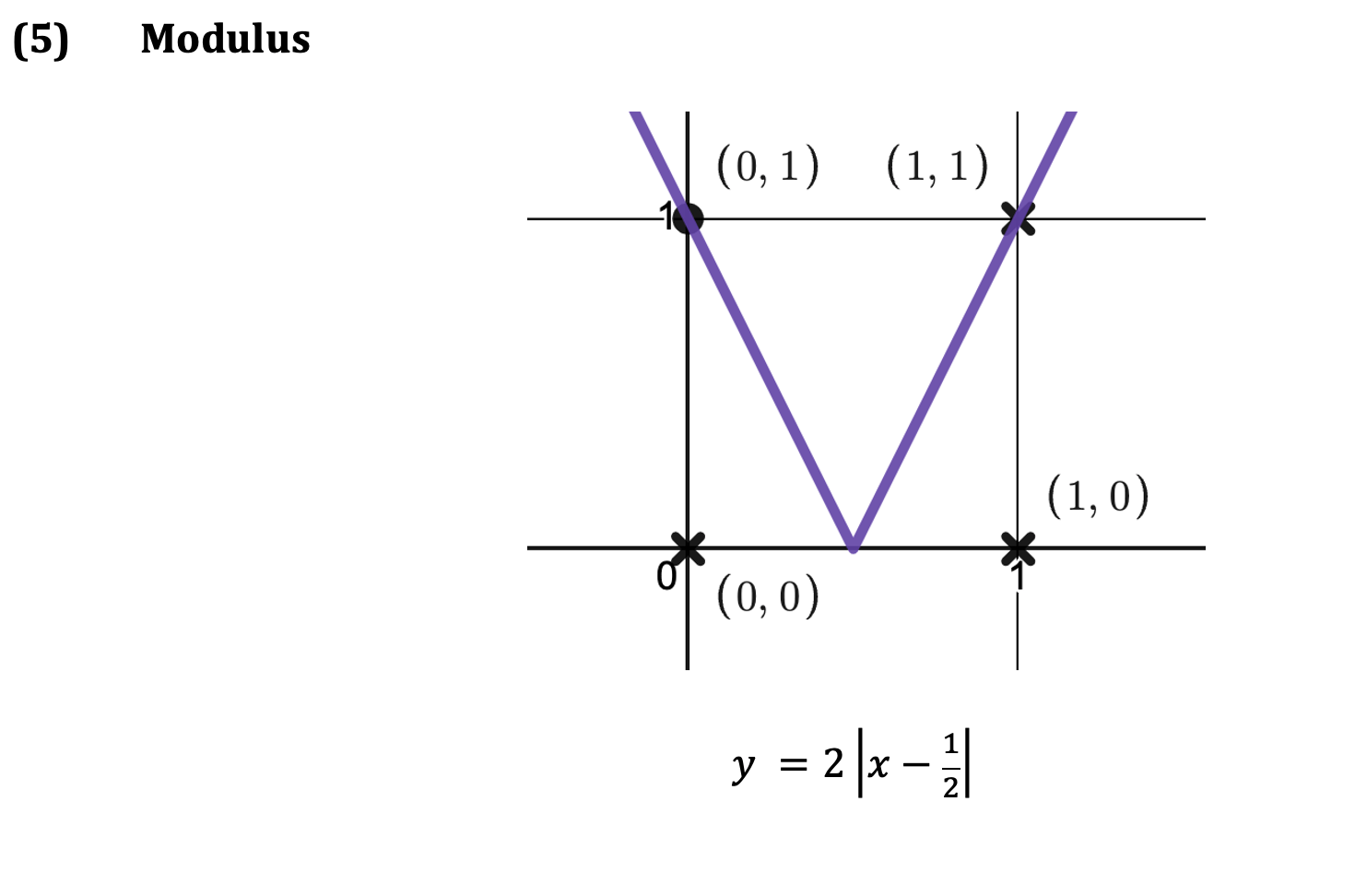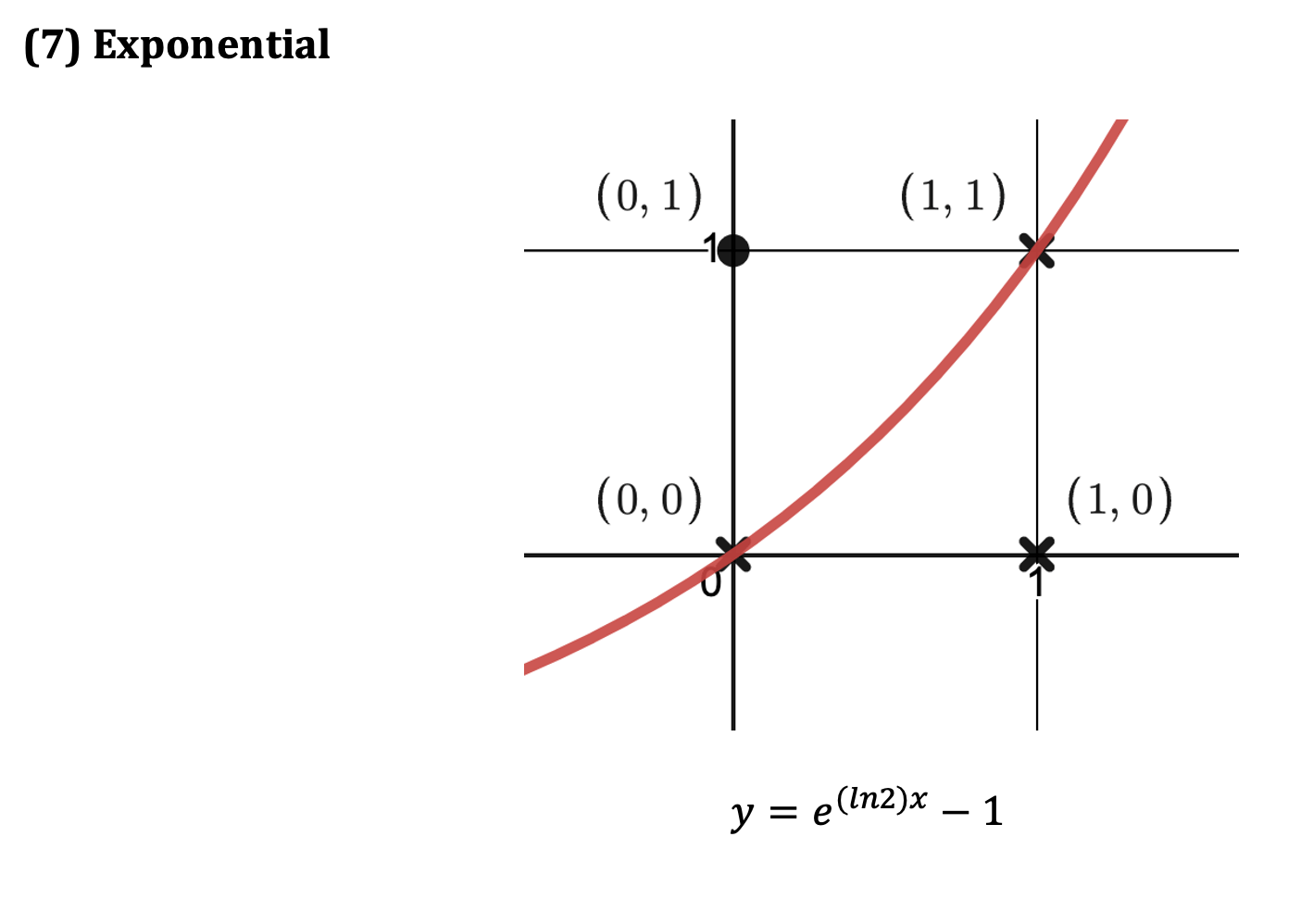You can download a pdf of the problem and the solution here.